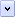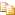JScript has a full range of operators, including arithmetic, logical, bitwise, assignment, as well as some miscellaneous operators.

# Computational Operators

Description Symbol

-

++

--

*

/

%

+

-

# Logical Operators

Description Symbol

!

<

>

<=

>=

==

!=

&&

||

?:

,

===

!==

# Bitwise Operators

Description Symbol

~

<<

>>

>>>

&

^

|

# Assignment Operators

Description Symbol

=

OP=

# Miscellaneous Operators

Description Symbol

delete

typeof

void

instanceof

new

in

The difference between == (equality) and === (strict equality) is that the equality operator will coerce values of different types before checking for equality. For example, comparing the string "1" with the number 1 will compare as true. The strict equality operator, on the other hand, will not coerce values to different types, and so the string "1" will not compare as equal to the number 1.

Primitive strings, numbers, and Booleans are compared by value. If they have the same value, they will compare as equal. Objects (including Array, Function, String, Number, Boolean, Error, Date and RegExp objects) compare by reference. Even if two variables of these types have the same value, they will only compare as true if they refer to exactly the same object.

For example:Copy Code
```// Two primitive strings with the same value.
var string1 = "Hello";
var string2 = "Hello";

// Two String objects, with the same value.
var StringObject1 = new String(string1);
var StringObject2 = new String(string2);

// This will be true.
if (string1 == string2)
// do something (this will be executed)

// This will be false.
if (StringObject1 == StringObject2)
// do something (this will not be executed)

// To compare the value of String objects,
// use the toString() or valueOf() methods.
if (StringObject1.valueOf() == StringObject2)
// do something (this will be executed)```

Home